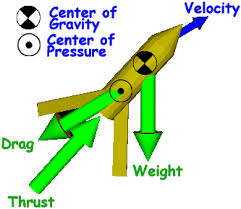Published on Mar 28, 2020

Abstract

The objective:

The purpose of this project was to find out which of the shapes, rectangle, parallelogram, triangle, trapezoid, and elliptical, caused a model rocket to reach the highest altitude (apogee).

Methods/Materials

In my study, I built five rockets each with the same weight, shape, dimensions, and engine. The only difference between the rockets tested were the geometrical shape of the fins, but the area of all the fin shapes were the same.

Each rocket was launched several times with an altimeter in the payload section in order to record the highest reached altitude of every flight. The altitude (dependent variable) that each rocket will reach will depend on the fin shape (independent variable).Results

The results of my experiment show that the elliptical fin design is the best fin design, with a maximum apogee of 961 feet and an average apogee of 949 feet.

The rectangular fin design came in second place with a maximum apogee of 878 feet and an average apogee of 838 feet. The third best fin design was the parallelogram, with a maximum apogee of 861 feet and an average apogee of 823 feet.

The triangular fin design came in fourth with a maximum apogee of 834 feet and an average apogee of 817 feet. The least successful fin design tested in my experiment was the trapezoidal design.

It came in last with a maximum apogee of 820 feet and an average apogee of 810 feet.

Conclusions/Discussion

Through this experiment, we can conclude that the best possible fin design is the elliptical. My study proved that my hypothesis was correct in that if I launched five rockets each with a different fin shape (rectangle, trapezoid, triangle, elliptical (half circle), and parallelogram), then the rocket with the elliptical (half circle) shaped fins would reach the highest altitude.

The reason I think that the elliptical fin design had the best results is because its curved design cuts through the air more smoothly, and so creates less air turbulents and has less drag on the rocket.If I were to do this project again, I would test more unique and complex fin designs.

This project tests which fin shape (elliptical, parallelogram, rectangle, trapezoid, and triangle) causes a model rocket to reach the highest altitude, while maintaining all other aspects of the rocket the same.